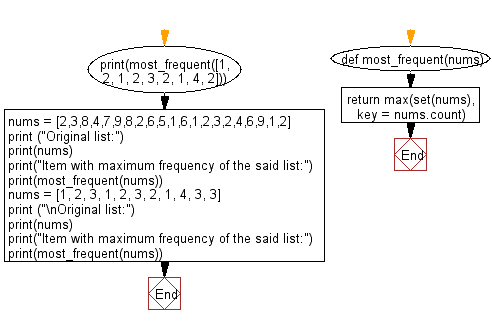﻿ Python: Most frequent element in a given list of numbers - w3resource# Python: Most frequent element in a given list of numbers

## Python List: Exercise - 261 with Solution

Write a Python program to get the most frequent element in a given list of numbers.

• Use set() to get the unique values in nums.
• Use max() to find the element that has the most appearances.

Sample Solution:

Python Code:

``````def most_frequent(nums):
return max(set(nums), key = nums.count)
print(most_frequent([1, 2, 1, 2, 3, 2, 1, 4, 2]))
nums = [2,3,8,4,7,9,8,2,6,5,1,6,1,2,3,2,4,6,9,1,2]
print ("Original list:")
print(nums)
print("Item with maximum frequency of the said list:")
print(most_frequent(nums))
nums = [1, 2, 3, 1, 2, 3, 2, 1, 4, 3, 3]
print ("\nOriginal list:")
print(nums)
print("Item with maximum frequency of the said list:")
print(most_frequent(nums))
```
```

Sample Output:

```2
Original list:
[2, 3, 8, 4, 7, 9, 8, 2, 6, 5, 1, 6, 1, 2, 3, 2, 4, 6, 9, 1, 2]
Item with maximum frequency of the said list:
2

Original list:
[1, 2, 3, 1, 2, 3, 2, 1, 4, 3, 3]
Item with maximum frequency of the said list:
3
```

Flowchart:## Visualize Python code execution:

The following tool visualize what the computer is doing step-by-step as it executes the said program:

Python Code Editor:

Have another way to solve this solution? Contribute your code (and comments) through Disqus.

What is the difficulty level of this exercise?

Test your Python skills with w3resource's quiz

﻿

## Python: Tips of the Day

Floor Division:

When we speak of division we normally mean (/) float division operator, this will give a precise result in float format with decimals.

For a rounded integer result there is (//) floor division operator in Python. Floor division will only give integer results that are round numbers.

```print(1000 // 300)
print(1000 / 300)```

Output:

```3
3.3333333333333335```